# 101

## Summary

### Factorization

The number 101 is a prime number.

## Polynomials

### Prime-generating polynomials

Below are some polynomials that give prime numbers for small input values, which give the value 101 for suitable input choice.

Polynomial Degree Some values for which it generates primes Input valueat which it generates 1012 all numbers 1-10, because 11 is one of the lucky numbers of Euler. 10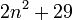2 all numbers 0-28 6

### Irreducible polynomials by Cohn's irreducibility criterion

By Cohn's irreducibility criterion, we know that if we write 101 in any base greater than or equal to 2, the corresponding polynomial is irreducible. We list here the irreducible polynomials of this type that are of degree at least two:

Base101 in baseCorresponding irreducible polynomial
2 1100101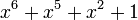3 102024 1211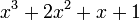5 401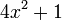6 245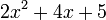7 203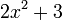8 1459 122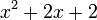10 101